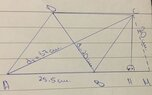# Parallelogram with diagonals. Need to find the area (S).

• MHB
• STS

#### STS

diagonal 1=20cm.
diagonal 2=37cm.
AB=25.5cm

S (AMC)= 306cm.
S (ABCD)=?

diagonal 1=20cm.
diagonal 2=37cm.
AB=25.5cm
Okay, that makes sense.

S (AMC)= 306cm.
S (ABCD)=?
What?? What is "S( )"? What is "M"? Is it another point? The midpoint where the two diagonals intercept?

#### Attachments

•333741DD-913B-4F0E-971E-5FF0F2D26C04.jpeg
11.5 KB · Views: 28
Last edited:
Okay, that makes sense.

What?? What is "S( )"? What is "M"? Is it another point? The midpoint where the two diagonals intercept?

S is the area. You move one of the diaganals to the side, then that forms a triangle. Then with Heron's formula you figure out the area of the triangle that has formed (AMC). That is suppose to help you figure out the area of the parallelogram using another formula, but I couldn't figure it out.

S is the area. You move one of the diaganals to the side, then that forms a triangle. Then with Heron's formula you figure out the area of the triangle that has formed (AMC). That is suppose to help you figure out the area of the parallelogram using another formula, but I couldn't figure it out.
OK; then WHY did you post only this:
........
diagonal 1=20cm.
diagonal 2=37cm.
AB=25.5cm

S (AMC)= 306cm.
S (ABCD)=?
........

You're given the 2 diagonals plus 1 side.
That's plenty of info to calculate the area.
S(AMC) = 306 not required...